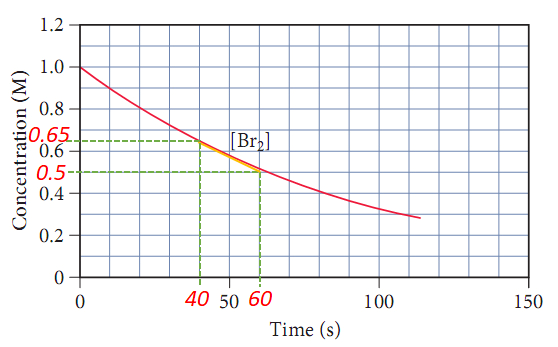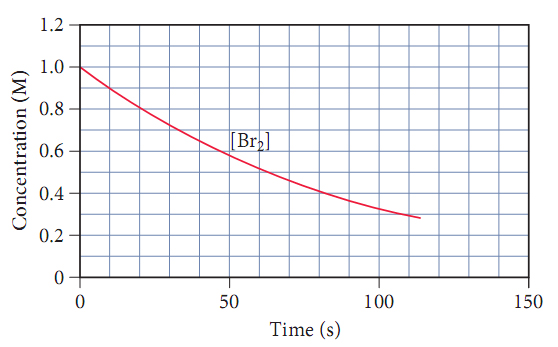# Problem: Consider the following reaction: H2(g) + Br2(g) → 2HBr. The graph below shows the concentration of Br2 as a function of time.Use the graph to calculate the instantaneous rate of formation of HBr at 50 s.

🤓 Based on our data, we think this question is relevant for Professor Khalil's class at UW-SEATTLE.

###### FREE Expert Solution

An instantaneous reaction rate is the rate at a particular moment in the reaction and is usually determined graphically (slope of tangent line) and can be calculated as:

Δ[  ] = change in concentration
Δt = change in time###### Problem Details

Consider the following reaction: H2(g) + Br2(g) → 2HBr. The graph below shows the concentration of Br2 as a function of time.Use the graph to calculate the instantaneous rate of formation of HBr at 50 s.